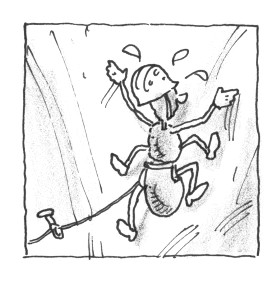### Home > CALC > Chapter 12 > Lesson 12.1.1 > Problem12-8

12-8.

A bug is climbing up a wall. When she starts out, she has the energy to move up quickly, but as time passes she tires, and moves more and more horizontally on the wall, which is less work than going up. These trends are captured by the derivative equations: $\frac { d y } { d t } = t - \frac { t ^ { 2 } } { 10 } , \frac { d x } { d t } = \operatorname { ln } ( t + 1 )$ where $t$ is measured in minutes and distance in feet. How far does the bug travel along the wall in the first 10 minutes?

$\int_0^{10}\sqrt{(\ln(t+1))^2+\Big(t-\frac{t^2}{10}\Big)^2}dt$